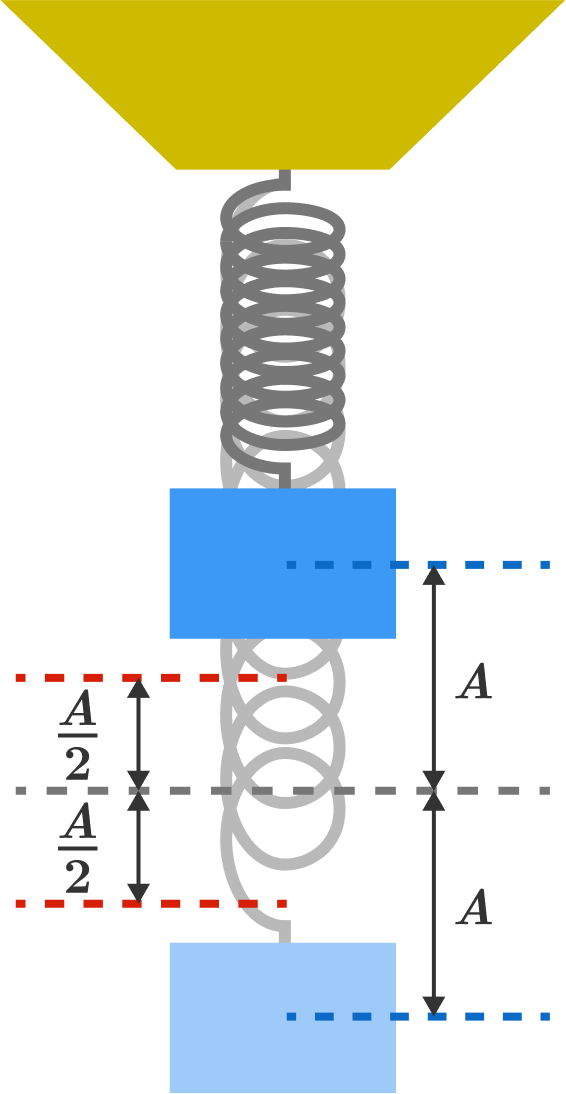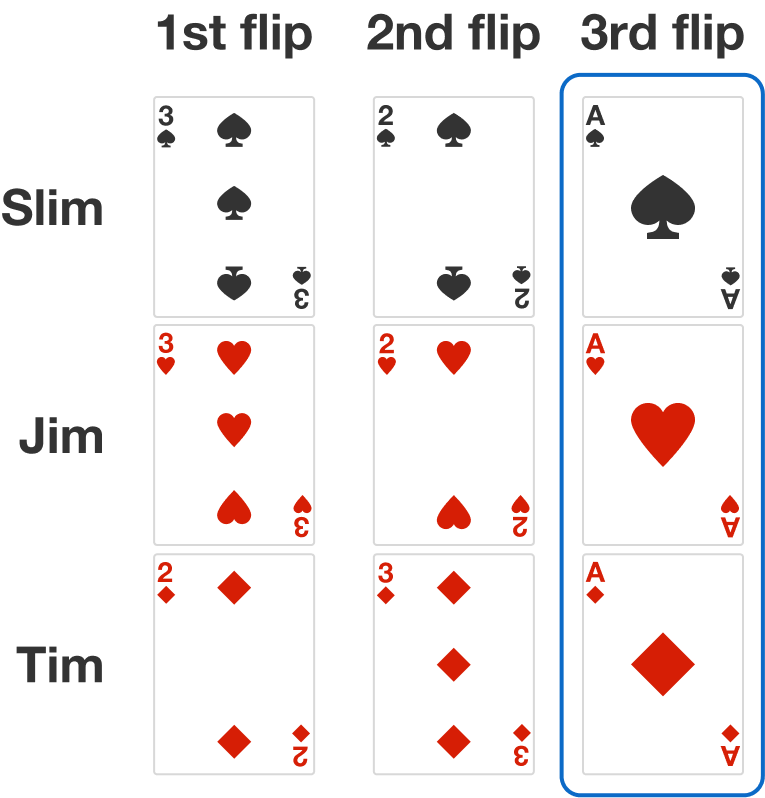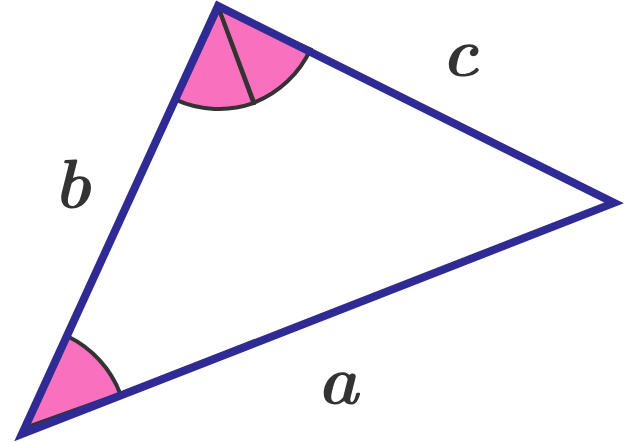# Problems of the Week

Contribute a problem

# 2018-10-22 IntermediateThis block oscillates harmonically with amplitude $A.$

What is the probability of finding the block's center of mass between positions $-\frac{A}{2}$ and $\frac{A}{2}?$

According to the exponent tower rule, $x^{(x^x)} = (x^x)^x$ is generally not true. However, this equality holds for some $x>0.$

What is the sum of all such $x$'s?

The equation $x^2 - ax + 1 = 0$ has real solutions, the greater of which is $r.$

Now, let $b$ be a positive real number such that the greater solution of the equation $x^2 - bx + 1 = 0$ is $\sqrt{r}.$

If $a = 79,$ then what is $b?$

Three friends, Slim, Jim, and Tim, each hold three cards—an ace, a two, a three—in a random order.

There are three turns and, on each turn, they simultaneously flip over a card face up, as illustrated below (without returning the card to their hands).

The probability that there's a three-way match on at least one of the flips is $\frac{a}{b},$ where $a$ and $b$ are coprime positive integers.

What is $a+b?$The three-way match can be on any of the flips, and can be on more than one flip. In this example, there is a three-way match on the third flip.The sides of a triangle are in an arithmetic progression, and the greatest angle of the triangle is double the smallest angle.

The ratio of the three sides can be expressed as $a:b:c,$ where $a,b,c$ are coprime positive integers.

What is $a + b + c ?$

×